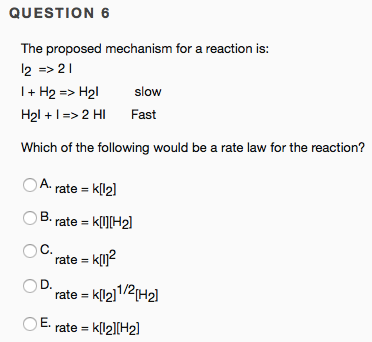# The proposed mechanism for a reaction is: I2 => 2 I I + H2 => H2I slow H2I + I => 2 HI fast Which of the following would be a rate law for the reaction? a) rate = k[I2] b) rate = k[I][H2] c) rate = k[I]^2 d) rate = k[I2]^1/2[H2] e) rate = k[I2][H2]